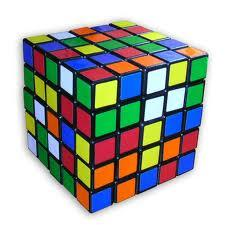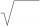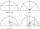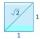# Transforming cuboid

Cuboid with dimensions 6 cm, 10, and 11 cm is converted into a cube with the same volume. What is its edge length?

Correct result:

x =  8.7 cm

#### Solution:We would be pleased if you find an error in the word problem, spelling mistakes, or inaccuracies and send it to us. Thank you!Tips to related online calculators
Looking for a statistical calculator?
Do you want to convert length units?
Tip: Our volume units converter will help you with the conversion of volume units.

## Next similar math problems:

• LogsTrunk diameter is 52 cm. Is it possible to inscribe a square prism with side 36 cm?
• Expression 6Evaluate expression: -6-2(4-8)-9
• RootsCalculate the square root of these numbers:
• Numeric questionIt is possible negative irrational number?
• NumberIs number 5.146852 irrational?